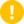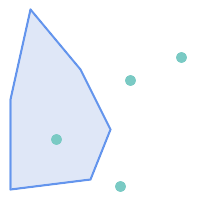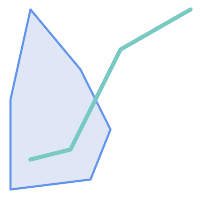## Name

ST_Crosses — Tests if two geometries have some, but not all, interior points in common

## Synopsis

`boolean ST_Crosses(`geometry g1, geometry g2`)`;

## Description

Compares two geometry objects and returns `true` if their intersection "spatially crosses"; that is, the geometries have some, but not all interior points in common. The intersection of the interiors of the geometries must be non-empty and must have dimension less than the maximum dimension of the two input geometries, and the intersection of the two geometries must not equal either geometry. Otherwise, it returns `false`. The crosses relation is symmetric and irreflexive.

In mathematical terms: ST_Crosses(A, B) ⇔ (dim( Int(A) ⋂ Int(B) ) < max( dim( Int(A) ), dim( Int(B) ) )) ∧ (A ⋂ B ≠ A) ∧ (A ⋂ B ≠ B)

Geometries cross if their DE-9IM Intersection Matrix matches:

• `T*T******` for Point/Line, Point/Area, and Line/Area situations

• `T*****T**` for Line/Point, Area/Point, and Area/Line situations

• `0********` for Line/Line situations

• the result is `false` for Point/Point and Area/Area situationsThe OpenGIS Simple Features Specification defines this predicate only for Point/Line, Point/Area, Line/Line, and Line/Area situations. JTS / GEOS extends the definition to apply to Line/Point, Area/Point and Area/Line situations as well. This makes the relation symmetric.This function automatically includes a bounding box comparison that makes use of any spatial indexes that are available on the geometries.Enhanced: 3.0.0 enabled support for `GEOMETRYCOLLECTION`This method implements the OGC Simple Features Implementation Specification for SQL 1.1.

s2.1.13.3This method implements the SQL/MM specification.

SQL-MM 3: 5.1.29

## Examples

The following situations all return `true`.`MULTIPOINT` / `LINESTRING``MULTIPOINT` / `POLYGON``LINESTRING` / `POLYGON``LINESTRING` / `LINESTRING`

Consider a situation where a user has two tables: a table of roads and a table of highways.

 ```CREATE TABLE roads ( id serial NOT NULL, geom geometry, CONSTRAINT roads_pkey PRIMARY KEY (road_id) );``` ```CREATE TABLE highways ( id serial NOT NULL, the_gem geometry, CONSTRAINT roads_pkey PRIMARY KEY (road_id) );```

To determine a list of roads that cross a highway, use a query similiar to:

```SELECT roads.id